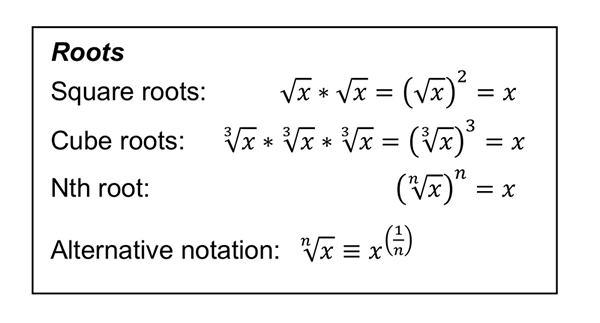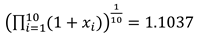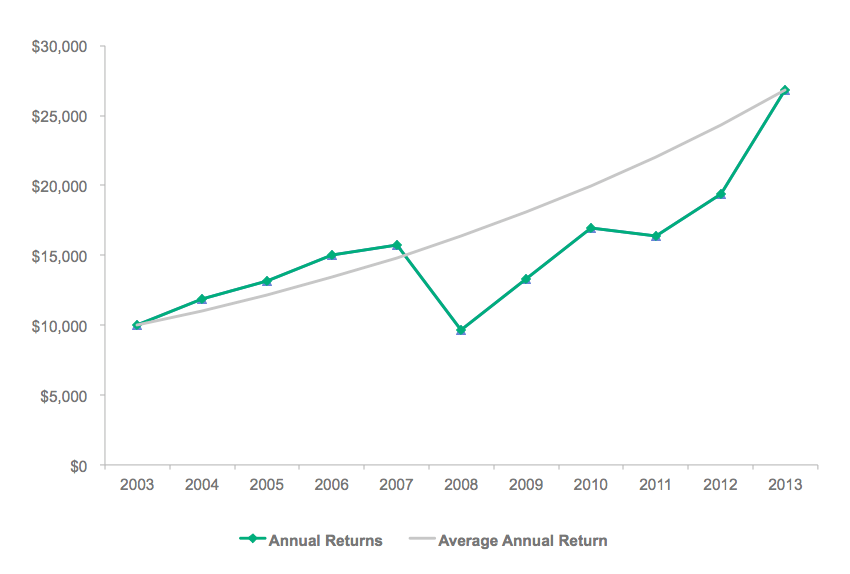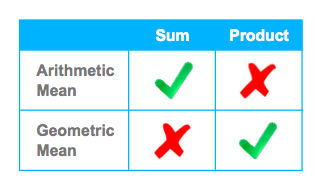Practical analysis for investment professionals
23 March 2015

# The Myth of Volatility Drag (Part 1)Whenever the market has volatility we lose money. We call this ‘volatility drag.’”

“Price fluctuations create a continual drag on your portfolio growth.”

“The more volatile our year-to-year returns, the less money we actually have. . . . We call this the ‘volatility drag.’ I also like to call it the volatility tax.”

“An investment with 10% volatility faces a drag on return of 0.50%. . . . The drag grows quickly as volatility increases.”

Scary stuff! But reading these articles, I found myself wondering, is it actually true? Like so many myths, there is a kernel of truth. But the interpretation that volatility is an active force, “pulling” down returns and costing you money, is simply not correct.

In Part 1, we will explore the crux of the issue, understanding the geometric mean. In Part 2, we will apply this understanding to arguments for volatility drag.

Where—Force Art Thou?

Remember free-body diagrams from physics class, the drawings of an object with arrows representing the forces? Drag was a common force, present when an object moved through a medium like air or water. So where does the “volatility drag” force come from? What medium is it that prices move through?

The proposition is that the difference between the geometric mean and the arithmetic mean of a series of returns represents “drag.” Let us revisit the geometric mean, and perhaps we can find a force. (The geometric mean is often encountered in the form of compound annual growth rate (CAGR). CAGR is a special case of geometric mean, useful when the initial value and ending value for n periods are known.)

To motivate the discussion, let’s look at an example of past performance data on a mutual fund prospectus that makes use of the geometric mean to calculate the “average annual total return.”

Average Annual Total Return

If you have read a mutual fund prospectus, then you have seen a chart similar to the following one, showing the annual total returns for the previous 10 years.

Annual Total Returns — Vanguard Extended Market Index Fund Institutional SharesThe percent returns are the year-over-year numbers and are straightforward. These charts are accompanied by a table that includes average annual total returns for one, five, and 10 years.

Average Annual Total Returns for Periods Ended 31 December 2013Here we must be a little cautious: This use of average is not the most common usage. In most cases, when we see the term average, we add up the numbers and divide the total by the number of elements. A quick check with the five-year return before taxes, calculating the usual average, is 23.75%. But the table says 22.68%. That is because there is more than one type of average, or mean, and in this case the geometric mean is the appropriate one to use. So why is it “appropriate”?

Means: A Quick Refresher

The arithmetic mean is the one with which we are most familiar. It is a property of sums: 2 + 8 is a sum of two elements. The one value that relates the number of elements to the sum of the elements is the arithmetic mean.

2 + 8 = 10 and the arithmetic mean is 10/2 = 5

The geometric mean is the analogous property for products. 2 * 8 is a product of the same two elements. The value that relates the number of elements to the product of the elements is the geometric mean.

2 * 8 = 16: Is there one number that when multiplied by itself equal 16?

Yes, and we can find it with the square root. √16 = 4. This is the geometric mean.

What if we had three elements? Say 2 + 8 + 32= 42. The arithmetic mean is 42/3 = 14.

Now try 2 * 8 * 32 = 512. We have three numbers, so need a cubed root: ∛512 = 8In general, the arithmetic mean is defined as:The geometric mean is defined as:Back to the Prospectus

Another chart you may see on a prospectus or fact sheet is the growth of a hypothetical investment using the annual returns.

Hypothetical \$10,000 InvestmentCan we calculate the final value using only the returns?

The initial value multiplied by 1 plus each of the annual returns in decimal form will get us there. (For more information on where the 1 came from and on logarithms in general, this article provides a good primer.)

This Is a Product, Not a Sum

\$10,000 * 1.1892 * 1.105 * 1.1446 * 1.0451 * 0.6142 * 1.3769 * 1.2759 * .9643 * 1.1850 * 1.3842 = \$26,828

As we discussed in the section above, the geometric mean is the average to use on a product. So, the geometric mean of the returns xi over 10 years isor a 10.37% annualized return, which matches what we were given in the table! With this value, we can calculate the ending value directly: \$10,000 * 1.1037^10 = \$26,828. The average annual return also lets us calculate the value for the intermediate years, as if there was an investment that earned exactly the average annual return every year. This is plotted below, showing a smooth curve that starts and ends at the same points.

Hypothetical \$10,000 InvestmentWhat about Log Returns, Are They Related?

Yes! One property of logarithms is that they transform a product to a sum: log(a * b * c) = log(a) + log(b) + log(c). Once transformed to a sum, the arithmetic mean applies. Taking the arithmetic mean of the log returns and then converting it back to the standard form is equivalent to the geometric mean. (In this context, a, b, and c would represent “1 + return.” We will call “log(1+return)” the “log return.”)

This is a regularly used approach, sometimes mandated by regulators, to calculate average periodic returns. Using the previous set of returns and calculating their log-returns (using log10):Noting that 10.37% is the same value we determined above, we can see the two approaches are equivalent.

Summing Up

Now when statements are made regarding the average return, we can ask a few questions. What kind of average? If it’s from a series of chained returns (i.e., a product), are they talking about arithmetic mean of log returns? Are they talking about the geometric mean of the returns? If neither of these is the case, we must examine the conclusions more closely.“Volatility drag” is one such conclusion. We found that the arithmetic and geometric means are related — both are equations using the same set of numbers — but the difference between them is in the definition, not from a force. Why, then, is this such a persistent myth? There are two seductive arguments made in its favor. One is very simple and appeals to our intuition, but contains a flawed assumption. The other stems from a slight misinterpretation of terms in a widely used mathematical model of prices.

Using the language of arithmetic and geometric means developed here, we will look at these two arguments in the next post and consider some of the practical ramifications.

All posts are the opinion of the author. As such, they should not be construed as investment advice, nor do the opinions expressed necessarily reflect the views of CFA Institute or the author’s employer.

Tags:

Share On
##### Will Morrison

Will Morrison is manager, business analytics and reporting, at CFA Institute. Previously, he was an engineer at GE, specializing in robotic path planning. Morrison has earned the Claritas Investment Certificate. He holds a BA in computer science from Goucher College, a BS in electrical engineering from the Johns Hopkins University, and a masters of systems engineering from the University of Virginia.

## 13 thoughts on “The Myth of Volatility Drag (Part 1)”

1.Tanveer Ahmad says:

Interesting

2.Guillaume Rabault says:

Hi Will,

I agree that this is a bit of a myth… I wrote this mathematical post on the subject. Perhaps you’ll find this usefull.

http://investmentmath.com/finance/2014/03/04/volatility-drag.html

Cheers,

Guillaume

3.Don Chambers says:

Your article makes a great point. Authors who think that volatility diminishes growth also tend to think that diversification enhances growth. This is known as “diversification return” and its purported benefits are a myth unless market prices are mean-reverting. The following paper may be helpful to some readers:
The Limitations of Diversification Return, The Journal of Portfolio Management Summer 2014, Vol. 40, No. 4: pp. 65-76.

4.Tony Cooper says:

Volatility drag is defined as the difference between arithmetic returns and geometric returns. It is appropriate to call it “volatility drag” because it is higher when volatility is higher.

It is not a myth. It is real. It is always positive or zero. Bigfoot is a myth. Sigma squared isn’t.

In the context of leveraged ETFs volatility drag WILL cost you returns. That cost is no myth. The drag increases with volatility. If you leverage too much the volatility drag will bring returns down to zero?

The difference between zero and positive returns is no myth.

1.steve michael says:

could you supply some support for your argument and why the author is wrong? all you do is refute his argument in an aggressive manner

returns may have negative serial correlation and that would support your argument……. and that does NOT refute what the author wrote.

5.Troy Rieck says:

I think it is fair to say that this topic is only discussed because our industry continues to use arithmetic returns in some of its models, simulations and projections. If we only spoke about returns appropriate for multi-periods calculations – ie geometric returns – then we would not have to reconcile between the two.

A related topic is the interaction between the returns generated by a portfolio and the cash inflows and outflows experienced by the portfolio. Professor Michael Drew and others have written extensively about this topic in the context of retirement incomes (e.g. Sequencing risk). More volatile portfolio returns can increase the risk of running out of money before a given point in time.

6.Andy Behrens says:

Are you going to cover the volatility drag on the ability to spend out of a portfolio? That drag is real. Two return streams with the same CAGR and different levels of volatility can support different levels of spending.

7.tyc says:

I’m surprised to read a specific opinion in this topic. The result of a simple geometric return minus arithmetic return can only be between 0 and a +number. Ignore what volatility drag means for a moment and focus on the math, the result could be linear (like it or not negative numbers exit).

Someone mentioned about diversification return (DR). I don’t understand the reason for using variance as a substitution for return. If we applied what is stated above as the method to compute DR and treat the arithmetic computation as a benchmark, then DR is just the compounding differences between the two return calculation.

8.Bob Collie says:

An expansion of this argument is available here: http://www.russell.com/us/institutional-investors/research/the-volatility-paradox.page

9.Paul Bouchey says:

I can’t wait for Part 2, but the author should be careful as there is some debate in the literature on this issue. (For example, Chambers & Zdanowicz in the “Limitations of Diversification” paper in the JPM and my article “Volatility Harvesting” in the JWM.) It is true that the expected value of the portfolio is not reduced by volatility, but the expected value is a poor descriptor of the wealth distribution, which is highly skewed. The large outcomes on the upside skew the expected value. Everyone needs to go back and read Chapter 6 “Returns for the long run” by Markowitz in Portfolio Selection (1956). Take uncertainty out of the picture: imagine an investment that oscillates between +30% and -10% return with certainty. Average return = 10%. Growth rate = 8%. Volatility reduces the growth rate, relative to an investment that gave 10% each period with certainty.

10.Johan says:

When will part 2 be published?

11.kurt says:

no part 2? Perhaps it got squelched.

﻿